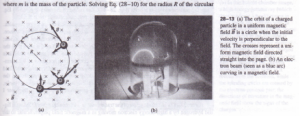# MOTION OF CHARGED PARTICLES IN A MAGNETIC FIELD

MOTION OF CHARGED PARTICLES IN A MAGNETIC FIELD

by Eq. (28-2), and the motion is determined by Newton’s laws. Figure 28-13 shows a simple example. A particle with positive charge q is at point 0, moving with in a uniform magnetic field directed into the plane of the figure. The are perpendicular, so the magnetic force F – qv x jj has magnitude F = quB and a direction as shown in the figure. The force is always perpendicular to v,so it can change the magnitude of the velocity, only its direction. To put it differently, the magnetic force never has a component parallel to the particle’s motion, so the magnetic force can never do work on the particle. This is true even if the magnetic field is not. Motion of a charged particle under the action of a magnetic field alone is ways motion with constant speed.

Using this principle, we see that in the situation shown in Fig. 28-13 a the magnitudes of both F and v are constant. At points such as P and S the directions of force and have changed as shown, but their magnitudes are the same. The particle therefore moves under the influence of a constant-magnitude force that is always at right angles to the velocity of the particle. Comparing these conditions with the discussion of circular motion in Sections 3-5 and 5-5, we see that the particle’s path is a circle, traced out with constant speed u (Fig. 28-13b). The centripetal acceleration is u2/R, and the only force acting is the magnetic force, so from Newton’s second law,The number of revolutions per unit time. This frequency f is independent the radius R of the path. It is called the cyclotron frequency; in a particle called a cyclotron, particles moving in nearly circular paths are given a boost twice revolution, increasing their energy and their orbital radii but not their angular velocity frequency. Similarly, a magneto, a common source of microwave radiation microwave ovens and radar systems, emits radiation with a frequency equal to the of circular motion of electrons in a vacuum chamber between the poles  magnet .  If the direction of the initial velocity is not perpendicular to the field, the component parallel to the field is constant because there is no force parallel to the Then the particle moves in a helix (Fig. 28-14). The radius of the helix is given by E.:: (28-11), where now v is the component of velocity perpendicular to the B field. Motion of a charged particle in a non-uniform magnetic field is more co Figure 28-15 shows a field produced by two circular coils separated by some dis Particles near either coil experience a magnetic force toward the center of the particles with appropriate speeds spiral repeatedly from one end of the region other and back. Because charged particles can be trapped in such a magnetic field, . called a magnetic bottle. This technique is used to confine very hot with of the order of 106 K. In a similar way the earth’s non-uniform magnetic traps charged particles coming from the sun in doughnut-shaped regions around earth, as shown in Fig. 28-16. These regions, called the Van Allen radiation were discovered in 1958 using data obtained by instruments aboard the satellite. .
Figure 28-17 a is a photograph of the track made in a bubble chamber filled . hydrogen by a high-energy electron moving in a magnetic field perpendicular